### Home > PC > Chapter 4 > Lesson 4.1.4 > Problem4-55

4-55.
1.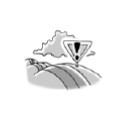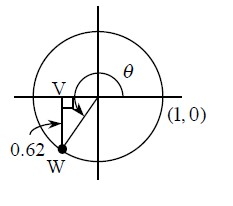Study the diagram of the unit circle below right, in which VW = 0.62. Homework Help ✎

1. Find sin θ.

2. Find cos θ.

3. α is another angle with the same sine as θ, but a different cosine. What quadrant is α in?

4. β is another angle with the same cosine as θ, but a different sine. What quadrant is β in?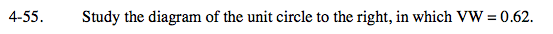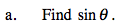In a unit circle, what is the radius?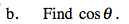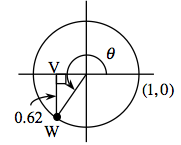You have two sides of a right triangle. Find the third side, which is the x-coordinate.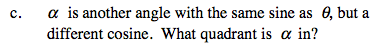In Quadrant III, sine and cosine are both negative.
In which quadrant is sine negative and cosine positive?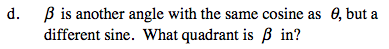In which quadrant is cosine negative and sine positive.